# Adding Mixed Numbers with Unlike DenominatorsTimes up!
Times up! You may finish answering the current question and then proceed to your score!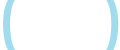Sorry, you have used all of your available hints for this lesson.
Practice Limit Reached

You've reached your daily practice limit of 12 questions.

Award InformationAwarded toQuestion HintChoose Family Member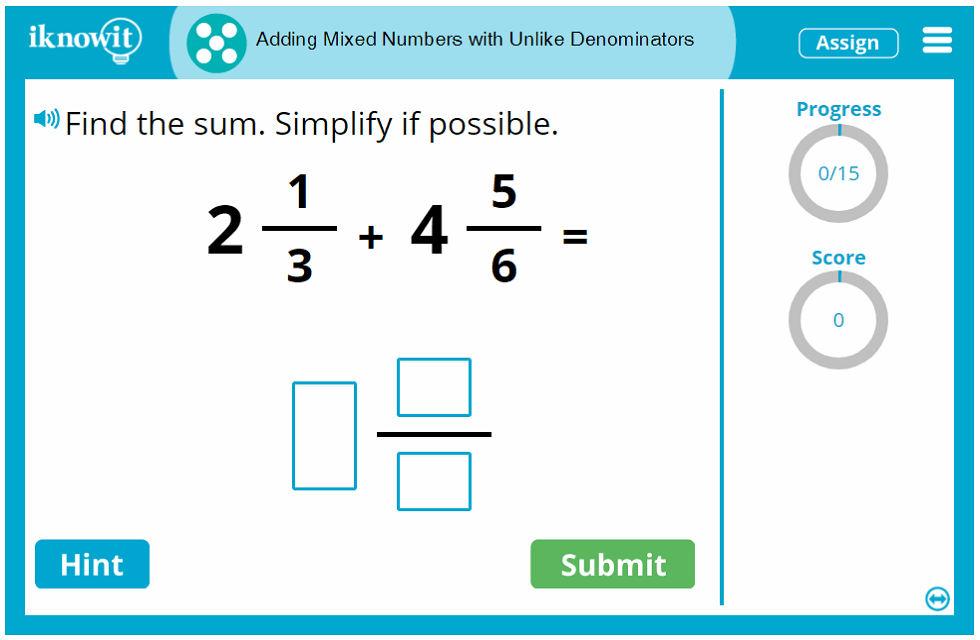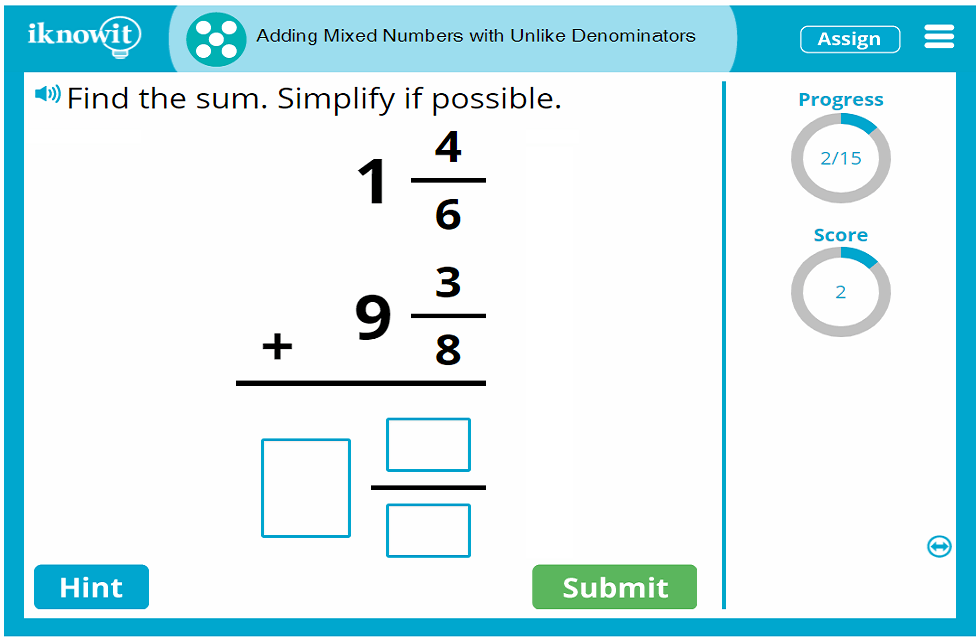## Online Math Game - Adding Mixed Numbers with Unlike Denominators

Your fifth grade students will practice adding mixed numbers with unlike denominators in this interactive, online math game from iKnowIt.com. This math practice activity will challenge students to attain the following learning objectives:

• Add mixed numbers with unlike denominators
• Find the common denominator
• Write equivalent fractions
• Simplify the fraction if possible

Students will be shown a variety of vertical addition problems and horizontal addition problems, as well as mixed number addition word problems. They will gain confidence and proficiency with adding mixed numbers with unlike denominators as they work through this fifth-grade math lesson.

As students practice addition with fractions in this online math activity, they will have several helpful tools available to them. If students get stuck on a fractions addition problem, for example, they can click on the "Hint" button, which will provide insight into the first step of the mixed number addition problem: "Find the common denominator. Write equivalent fractions." When students answer a question incorrectly, they will be shown a detailed explanation page with a step-by-step tutorial showing them how to solve the mixed fraction addition problem correctly. Students can move through their math practice session learning from prior mistakes.

Additional math lesson features help children make the most of their math practice session. For example, a progress-tracker in the upper-right corner of the practice screen shows students how many questions they have answered out of the total number of questions in the math lesson. A score-tracker beneath that lets students see how many points they have achieved for correct answers. Opposite, a speaker icon indicates the read aloud-feature; students can click this button to have the question read out-loud to them in a clear voice. This is an excellent option for ESL/ELL students and children who excel as auditory learners. Each one of the I Know It lesson features is designed with your students' math success in mind.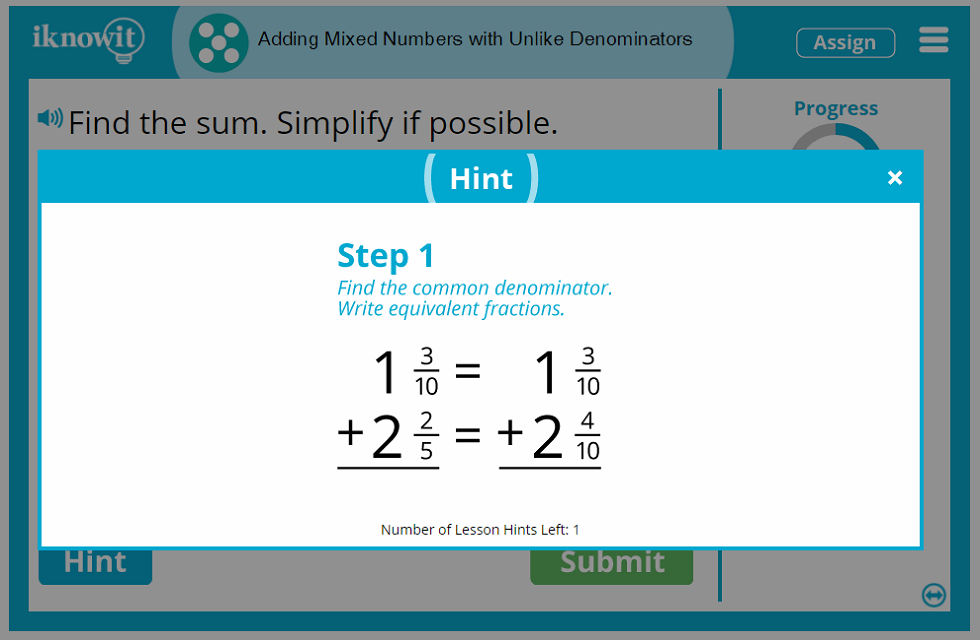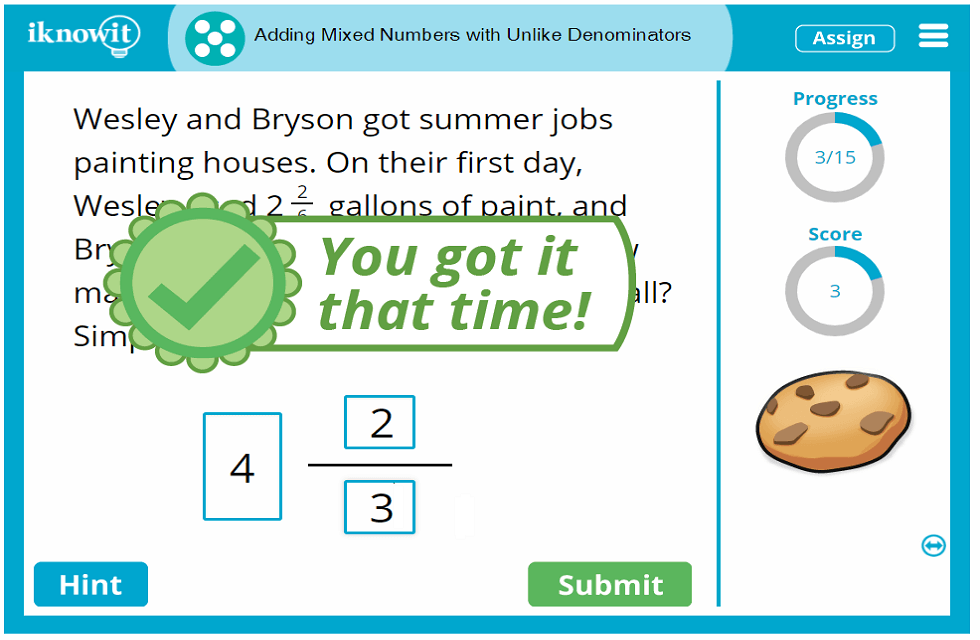## Strengthen Math Skills with Online Learning

Give your students an exciting, enriching way to practice math skills by using the I Know It online math practice program. Elementary teachers and homeschool educators alike enjoy using I Know It alongside a comprehensive math curriculum to encourage their students to reinforce important math concepts, from kindergarten through fifth grade. Teachers appreciate the wide array of math topics covered by our program. You can choose from dozens of math activities for each elementary grade level. Each math game has been written by accredited elementary math teachers to meet at least one Common Core Standard (often more). Math lessons are organized on the I Know It site by grade level and topic, making it easy to find and assign math practice activities in just a few clicks.

Students are delighted to practice math skills on iKnowIt.com. Kids get a kick out of the cute, animated characters waiting to cheer them on in their math practice activities with a silly dance or clever trick each time they solve a math problem correctly. Plenty of positive feedback messages encourage students to "Keep going!" even when they make mistakes. Plus, children can earn math awards for their virtual trophy case each time they master a new math milestone.

We hope you and your fifth-grade class will enjoy adding mixed numbers with unlike denominators in this interactive math lesson. Be sure to check out the hundreds of other fifth grade math practice lessons we have available on our website as well.

Children will log into iKnowIt.com using their unique login credentials. From the kid-friendly homepage, they will be able to locate the math activities you have assigned to them for practice. If you choose to give them the option in your administrator account, they can also explore other math activities at their grade level and beyond for extra review or an additional challenge. Grade levels are designated by letters (i.e., "Level E" for fifth grade), making it easy for you to assign math activities based on each child's needs and skill level.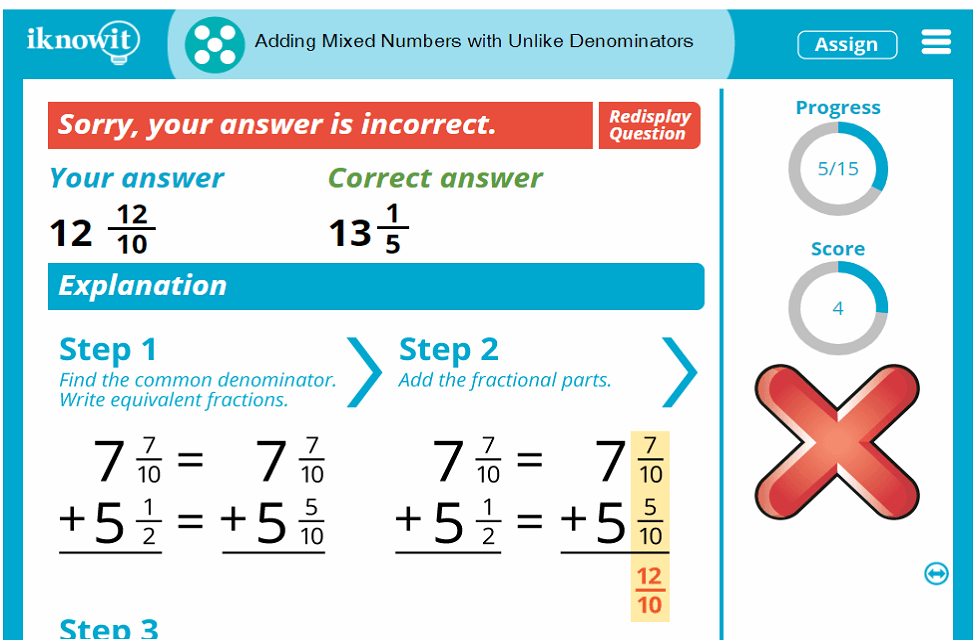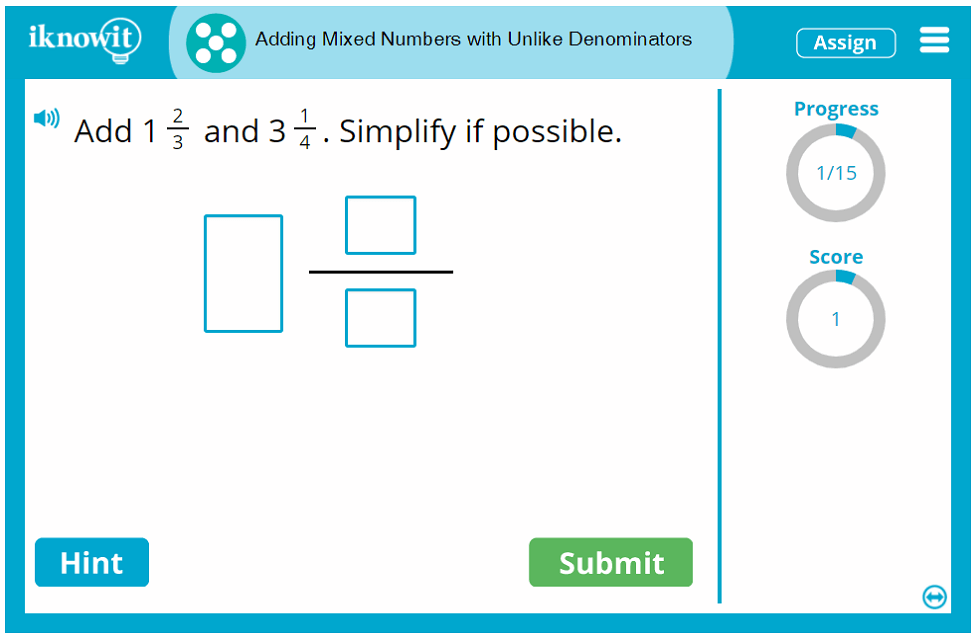## Level

This interactive math lesson is classified as Level E. It may be ideal for a fifth-grade class.

## Common Core Standard

5.NF.1
Number and Operations - Fractions

Students will use equivalent fractions as a strategy to add and subtract fractions. Students will add and subtract fractions with unlike denominators (including mixed numbers) by replacing given fractions with equivalent fractions in such a way as to produce an equivalent sum or difference of fractions with like denominators.

## You might also be interested in...

Adding Fractions with Unlike Denominators (Level E)
In this fifth grade-level math lesson, students will practice adding fractions with unlike denominators. Questions are presented as horizontal addition problems, vertical addition problems, and addition word problems.

Subtracting Mixed Numbers with Unlike Denominators (Level E)
In this math lesson geared toward fifth grade, students will practice subtracting mixed numbers with unlike denominators. Questions are presented as horizontal subtraction problems, vertical subtraction problems, and subtraction word problems.